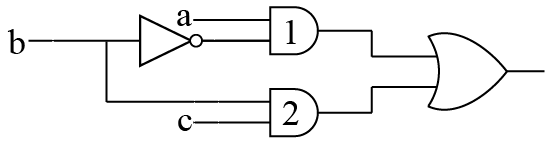# ISRO Scientist or Engineer Computer Science 2018

Instructions

For the following questions answer them individually

Question 21

#In the diagram above, the inverter (NOT gate) and the AND-gates labeled 1 and 2 have delays of 9, 10 and 12 nanoseconds(ns), respectively. Wire delays are negligible. For certain values of a and c, together with certain transition of b, a glitch (spurious output) is generated for a short time, after which the output assumes its correct value. The duration of the glitch isQuestion 22

# Which of the following comparisons between static and dynamic type checking is incorrect?Question 23

# ..................... can detect burst error of length less than or equal to degree of the polynomial and detects burst errors that affect odd number of bits.Question 24

# An array A consists of n integers in locations A[O], A ....A[n-1]. It is required to shift the elements of the array cyclically to the left by k places, where $$1 \leq k \leq (n-1)$$. An incomplete algorithm for doing this in linear time, without using another array is given below. Complete the algorithm byfilling in the blanks. Assume alt the variables are suitably declared.min = n ; i = 0;while (..........){ temp = A[i] ; j = i ; while (........){ A[j] = ...........; j = (j + k) mod n; if (j < min) then min = j ; } A[(n + i - k) mod n] = .............; i = ...........; }Question 25

# Station A uses 32 byte packets to transmit messages to Station B using a sliding window protocol. The round trip delay between A and is 80 ms and the bottleneck bandwidth on the path between A and B is 128 kbps. What is the optimal window size that A should use?Question 26

# Assuming that for a given network layer implementation, connection establishment overhead ts 100 bytes and disconnection overhead is 28 bytes. What would be the minimum size of the packet the transport layer needs to keep up, if it wishes to implement a datagram service above the network layer and needs to keep its overhead to a minimum of 12.5%. (Ignore transport layer overhead)Question 27

# In cryptography, the following usestransposition ciphers and the keyword is LAYER. Encrypt the following message. (Spaces are omitted during encryption)WELCOME TO NETWORK SECURITY !Question 28

# In a particular program, it is found that 1% of the code accounts for 50% of the execution time. To code a program in C++, it takes 100 man-days. Coding in assembly language ts 10 times harder than coding in C++, but runs 5 times faster. Converting an existing C++ program into an assembly lenguage program is 4 times faster.To completely write the program in C++ and rewrite the 1% code in assembly language, if a project team needs 13 days, the team consists ofQuestion 29

# Incremental-Compiler is a compilerQuestion 30

# DU-chains(Definition-Use) in compiler designOR